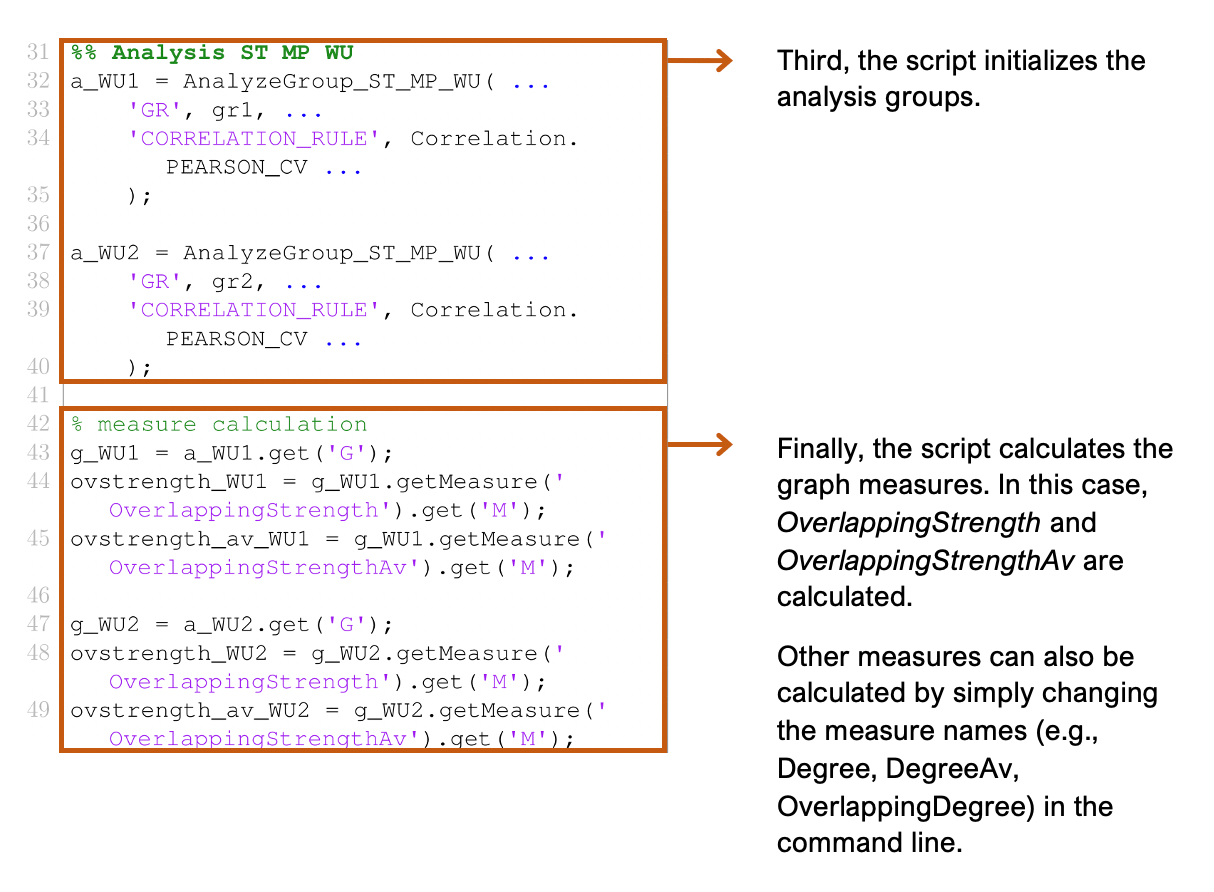# Calculating Different Measures

Description:

This is the developer tutorial for adapting a script for calculating different graph measures. In this tutorial, we will explain how to edit an example script to calculate different graph measures in braph2genesis. Here, we use EXAMPLE_ST_MP_WU as an example to show how to edit this script to calculate different graph measures for structural data using a multiplex weighted undirected graph.

File path:

\ braph2genesis \ pipelines \ structural multiplex \ example_ST_MP_WU.m